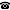# 2020 seminar talk: Local club condensation in extender models

Talk held by Gabriel Fernandes (Bar-Ilan University, Ramat Gan, Israel) at the KGRC seminar on 2020-11-19.

### Abstract

Local club condensation is a condensation principle defined by Friedman and Holy. It is a theorem due to Friedman and Holy that local club condensation holds in most of the extender models that are weakly iterable.

We prove that in any weakly iterable extender model with $\lambda$-indexing, given a cardinal $\kappa$, the sequence $\langle L_\alpha [E] \mid \alpha < \kappa^{++} \rangle$ witnesses local club condensation on the interval $(\kappa^+ , \kappa^{++})$ iff $\kappa$ is not a subcompact cardinal in $L[E]$.

We also prove that if $\kappa$ is subcompact, then there is no sequence $\langle M_\alpha \mid \alpha < \kappa^{++} \rangle \in L[E]$ with $M_\kappa = (H_\kappa)^{L[E]}$ and $M_{\kappa^{++}} = (H_{\kappa^{++}})^{L[E]}$ which witnesses local club condensation in $(\kappa^+ , \kappa^{++})$.

Using the equivalence between subcompact cardinals and $\neg\square_\kappa$, due to Schimmerling and Zeman, it follows that $\square_\kappa$ holds iff the sequence $\langle L_\alpha [E] \mid \alpha < \kappa^{++} \rangle$ witnesses local club condensation on the interval $(\kappa^+ , \kappa^{++})$.

### Time and Place

Talk at 3:00pm via Zoom

Kurt Gödel Research Center for Mathematical Logic. Währinger Straße 25, 1090 Wien, Austria.+43-1-4277-50501. Last updated: 2010-12-16, 04:37.